# 【老脸教你做游戏】小鸟飞过障碍物的游戏（下）

#### 阅读提示

• 想要学习利用游戏引擎开发游戏的朋友。本文不会涉及任何第三方游戏引擎。
• 不具备面向对象编程经验的朋友。本文的语言主要是Javascript(ECMA 2016)，如果你不具备JS编程经验倒也无妨，但没有面向对象程序语言经验就不好办了。#### 最基础的碰撞判定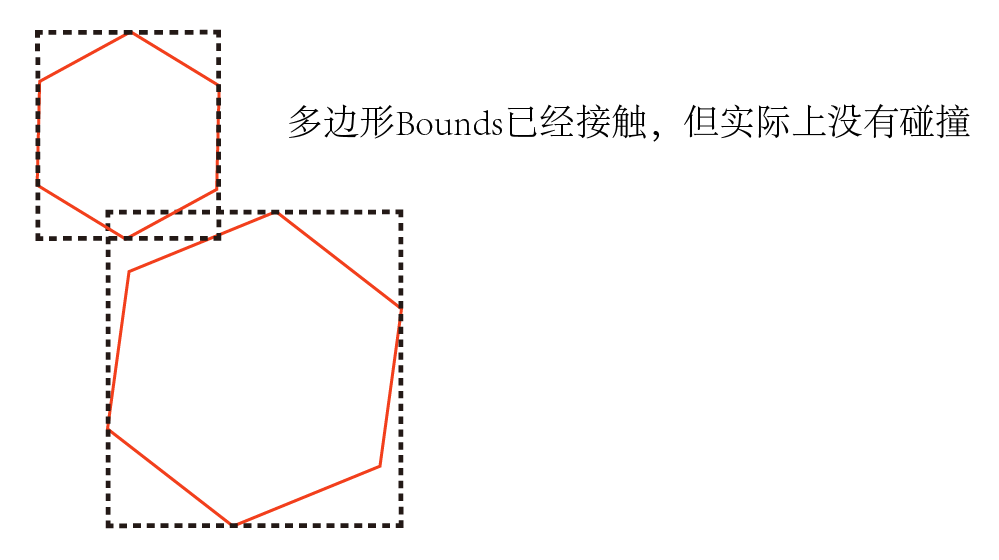function overlaps(boundsA,boundsB){
if(boundsA.left > boundsB.right
|| boundsA.top > boundsB.bottom){
return false;
}
if(boundsB.left > boundsA.right
|| boundsB.top > boundsA.bottom){
return false;
}
return true;
}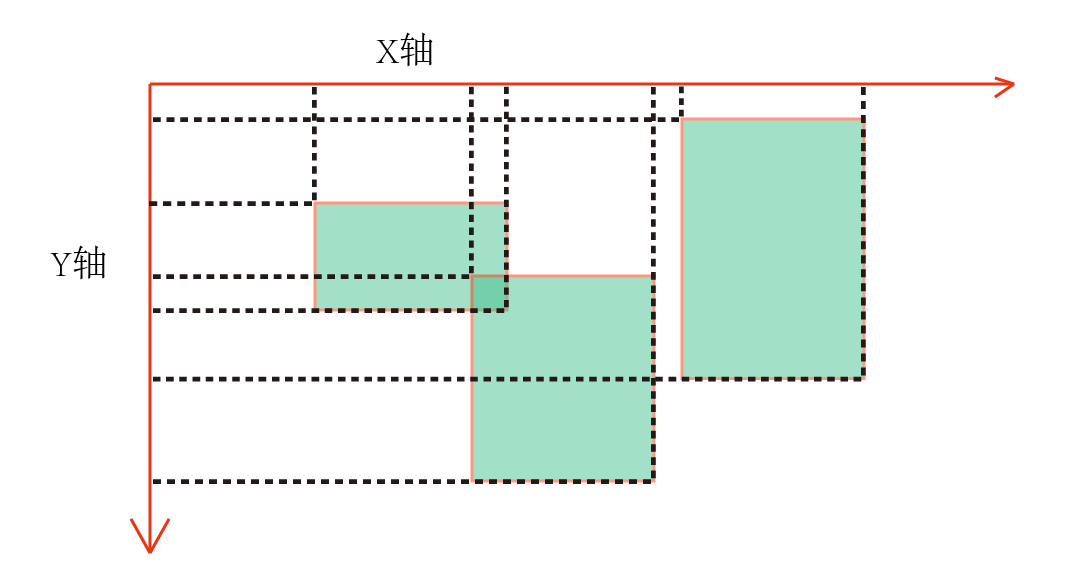function overlaps(boundsA, boundsB) {
return (boundsA.left <= boundsB.right && boundsA.right >= boundsB.left
&& boundsA.bottom >= boundsB.top && boundsA.top <= boundsB.bottom);
}



#### Figure类的第1次迭代

Figure.js :
...
getBounds() {
return {
left: this.left, top: this.top,
right: this.right, bottom: this.bottom
};
}

get right() {
return this.left + this.width;
}

get bottom() {
return this.top + this.height;
}
...


drawSelf(ctx) {
let imageManager = ImageManager.getInstance();
let trunk1 = imageManager.getImage('trunk1');
let trunk2 = imageManager.getImage('trunk2');
let trunk3 = imageManager.getImage('trunk3');
let startX = 0;
let startY = 0;
// 一列一列画：
for (let i = 0; i < this.mapData.mapData.length; i++) {
startY = 0;//每一列的y轴坐标都是从0开始
// 先计算出每一列绘制的起始x轴的值：
if (i != 0) {
// 从第二列开始就要计算了，第一列是0
startX += this.trunkWidth;// 第n列比第n-1列绝对多出一个宽度
// 再加上它们之间的间隔：
startX += this.mapData.spaceData[i-1] * this.trunkWidth;
}
// 某一列的地图数据：
let datas = this.mapData.mapData[i];
for (let j = 0; j < datas.length; j++) {
let data = datas[j];
let image = undefined;
switch (data) {
case 1:
image = trunk2;
break;
case 2:
image = trunk3;
break;
case 3:
image = trunk1;
break;

}
// 如果image为空，即地图数据为0，此处是个空白处就不需要绘制
if (image) {
ctx.drawImage(image, startX, startY, this.trunkWidth, this.trunkHeight);
}
// 画好一个就往下y坐标往下移动一个树桩高度
startY += this.trunkHeight;
}
}
}


import Figure from "./Figure";
import MapGenerator from "./MapGenerator";
import ImageManager from "./utils/ImageManager";

export default class TileMap extends Figure {
constructor(p) {
super(p);
this.mapData = undefined;
this.trunkHeight = 0; // 单个树桩的高度
this.trunkWidth = 0; // 单个树桩的宽度
this.column = 0;
this.row = 0;
this.boundsArray = []; // 地图中树桩的Bounds数据数组
}

initMap() {
if (this.mapData != undefined) {
MapGenerator.generateMapData(this.mapData);
} else {
this.mapData = MapGenerator.generateMapData(this.mapData, this.column, this.row);
}
// 根据map数据来设置Map的高度和宽度：
this.height = this.mapData.row * this.trunkHeight;
this.width = this.mapData.column * this.trunkWidth;
// 还要加上每列树桩之间的间隔：
for (let i = 0; i < this.mapData.spaceData.length; i++) {
this.width += this.mapData.spaceData[i] * this.trunkWidth;
}
// 生成树桩的bounds数据：
this.calculateTrunkBounds();
}

calculateTrunkBounds() {
let boundsArray = this.boundsArray;
boundsArray.length = 0;// 先清空
let startX = 0;
let startY = 0;
for (let i = 0; i < this.mapData.mapData.length; i++) {
startY = 0;//每一列的y轴坐标都是从0开始
// 先计算出每一列绘制的起始x轴的值：
if (i != 0) {
// 从第二列开始就要计算了，第一列是0
startX += this.trunkWidth;// 第n列比第n-1列绝对多出一个宽度
// 再加上它们之间的间隔：
startX += this.mapData.spaceData[i - 1] * this.trunkWidth;
}
// 某一列的地图数据：
let datas = this.mapData.mapData[i];
for (let j = 0; j < datas.length; j++) {
let data = datas[j];
if (data != 0) {
// 跳过缺口区域, bounds里还多了一个data属性
// 这是为了绘制的时候不必再从mapData里取数据判断树桩image了
boundsArray.push({
left: startX,
top: startY,
right: startX + this.trunkWidth,
bottom: startY + this.trunkHeight,
data: data
});
}
// y坐标往下移动一个树桩高度
startY += this.trunkHeight;
}
}
}

getTrunkBoundsArray() {
return this.boundsArray;
}

drawSelf(ctx) {
let imageManager = ImageManager.getInstance();
let trunk1 = imageManager.getImage('trunk1');
let trunk2 = imageManager.getImage('trunk2');
let trunk3 = imageManager.getImage('trunk3');
let trunkDatas = this.getTrunkBoundsArray();
for(let i = 0 ; i < trunkDatas.length;i++){
let trunkData = trunkDatas[i];
let image = undefined;
switch (trunkData.data) {
case 1:
image = trunk2;
break;
case 2:
image = trunk3;
break;
case 3:
image = trunk1;
break;
}
if (image) {
ctx.drawImage(image, trunkData.left, trunkData.top,
this.trunkWidth, this.trunkHeight);
}
}
}
}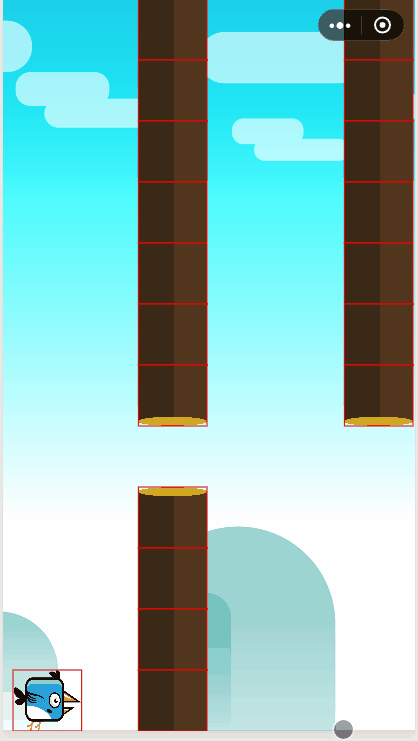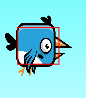BirdFlyGame.js部分代码：
....
afterRefresh(refreshCount) {
if(!this.gameOver){
this.collisionTest();
}
}
collisionTest() {
// 小鸟bounds：
let birdBounds = this.bird.getBounds();
let scaleW = this.bird.width * 0.2;
let scaleH = this.bird.height * 0.2;
// 让bounds缩小点
let a = {
left: birdBounds.left + scaleW,
top: birdBounds.top + scaleH,
right: birdBounds.left + (this.bird.width - scaleW),
bottom: birdBounds.top + (this.bird.height - scaleH)
};
let that = this;

collisionTrunkTest(this.firstMap);
collisionTrunkTest(this.secondMap);

function collisionTrunkTest(map){
let firstBounds = map.getTrunkBoundsArray();
for (let i = 0; i < firstBounds.length; i++) {
let bounds = firstBounds[i];
// bounds是相对地图的，需要加上它在Graph的位置
// 以此来获得树桩相对于Graph的Bounds
let b = {
left: bounds.left + map.left,
top: bounds.top + map.top,
right: bounds.right + map.left,
bottom: bounds.bottom + map.top
};
if(BirdFlyGame.overlaps(a,b)){
that.processGameOver();
return;
}
}
}
}
processGameOver(){
this.gameOver = true;
this.gameStop();
console.log('game over');
}
static overlaps(bounds1, bounds2) {
let a = bounds1;
let b = bounds2;
return (a.left <= b.right && a.right >= b.left
&& a.bottom >= b.top && a.top <= b.bottom);
}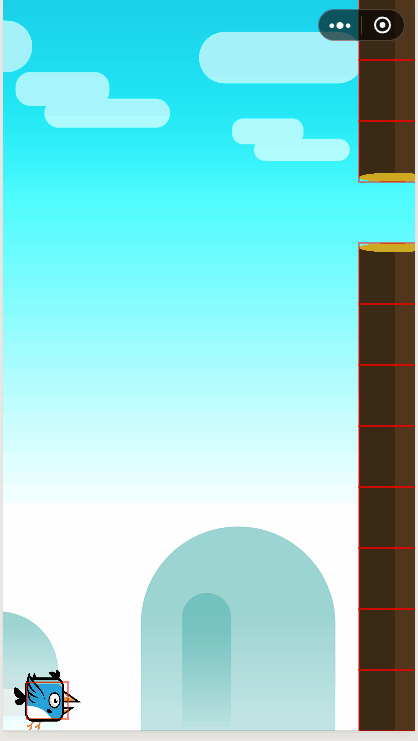#### 性能优化

##### 1.去掉不必要的绘制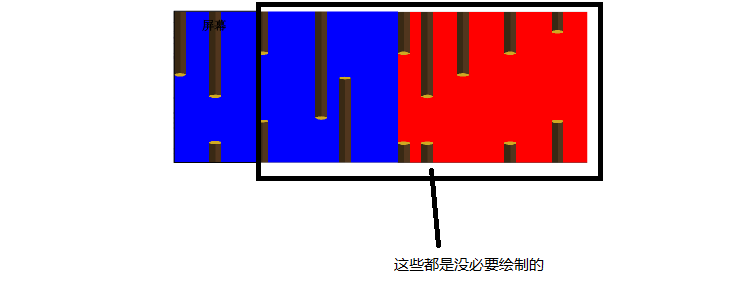drawChildren(ctx) {
for (let i = 0; i < this.children.length; i++) {
let childFigure = this.children[i];
// bounds碰撞判定基于Figure坐标系
// 所以Figure显示区域的bounds左上角就是[0,0]
let displayBounds = {left:0,top:0,
right:this.width,bottom:this.height};
if(!Utils.overlaps(displayBounds,childFigure.getBounds())){
continue;
}
childFigure.draw(ctx);
}
}


drawSelf(ctx) {
let imageManager = ImageManager.getInstance();
let trunk1 = imageManager.getImage('trunk1');
let trunk2 = imageManager.getImage('trunk2');
let trunk3 = imageManager.getImage('trunk3');
let trunkDatas = this.getTrunkBoundsArray();
let graph = this.getGraph();
let graphDisplayBounds = {
left: 0, top: 0,
right: graph.width,
bottom: graph.height
};
for (let i = 0; i < trunkDatas.length; i++) {
let trunkData = trunkDatas[i];
let image = undefined;
// 找到树桩对应graph坐标系的Bounds
let absoluteBounds = {
left: trunkData.left + this.left, top: trunkData.top + this.top,
right: trunkData.right + this.left, bottom: trunkData.bottom + this.top
};
// 不在显示区域就跳过这一个（如果像之前是个二维数组就好了，直接可以跳过一列）
if(!Utils.overlaps(absoluteBounds,graphDisplayBounds)){
continue;
}
switch (trunkData.data) {
case 1:
image = trunk2;
break;
case 2:
image = trunk3;
break;
case 3:
image = trunk1;
break;
}
if (image) {
ctx.drawImage(image, trunkData.left, trunkData.top,
this.trunkWidth, this.trunkHeight);
}
}
}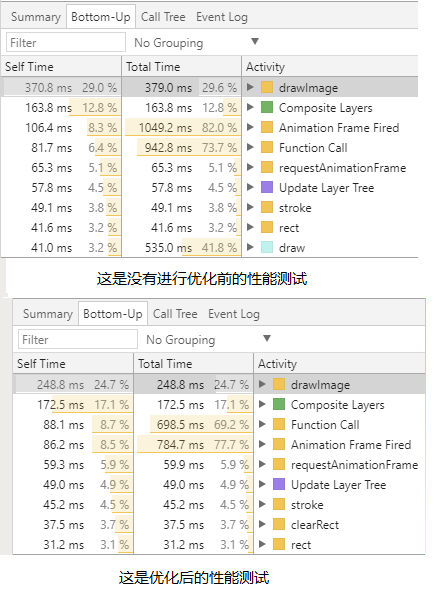##### 2.取消不必要的碰撞测试

collisionTrunkTest(this.firstMap);
collisionTrunkTest(this.secondMap);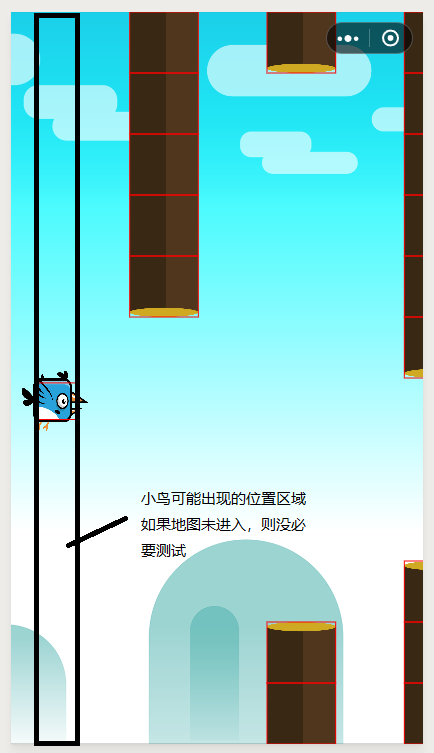之前存放的数据是这样的：

[{left:数字,top:数字,right:数字,bottom:数字,data:数字} , ........]

[
..........
// 某一列树桩bounds数据：
{
trunkBounds : [{left,top,right,bottom,data} , ........]
}
..........
]


BirdFlyGame.js ，修改碰撞测试方法：
collisionTest() {
// 小鸟bounds：
let birdBounds = this.bird.getBounds();
let scaleW = this.bird.width * 0.2;
let scaleH = this.bird.height * 0.2;
// 让bounds缩小点
let a = {
left: birdBounds.left + scaleW,
top: birdBounds.top + scaleH,
right: birdBounds.left + (this.bird.width - scaleW),
bottom: birdBounds.top + (this.bird.height - scaleH)
};
let that = this;
// 小鸟可能出现的区域bounds
let birdDisplayBounds = {
left : a.left,
right:a.right,
top:0,
bottom:this.height
};
collisionTrunkTest(this.firstMap);
collisionTrunkTest(this.secondMap);

function collisionTrunkTest(map){
if(!Utils.overlaps(map.getBounds(),birdDisplayBounds)){
// 如果地图没有进入小鸟出现区域，则不测试
return;
}
let firstBounds = map.getTrunkBoundsArray();
for (let i = 0; i < firstBounds.length; i++) {
let bounds = firstBounds[i];
// bounds是相对地图的，需要加上它在Graph的位置
// 以此来获得树桩相对于Graph的Bounds
let b = {
left: bounds.left + map.left,
top: bounds.top + map.top,
right: bounds.right + map.left,
bottom: bounds.bottom + map.top
};
if(Utils.overlaps(a,b)){
that.processGameOver();
return;
}else{
// 树桩和小鸟的bounds没有接触，看一下该bounds是否在小鸟bounds的右侧
// 如果是，则跳过剩余树桩的碰撞测试
if( b.left > a.right ) {
break;
}
}
}
}
}


## 再次感谢您的阅读！

#### 图形区域划分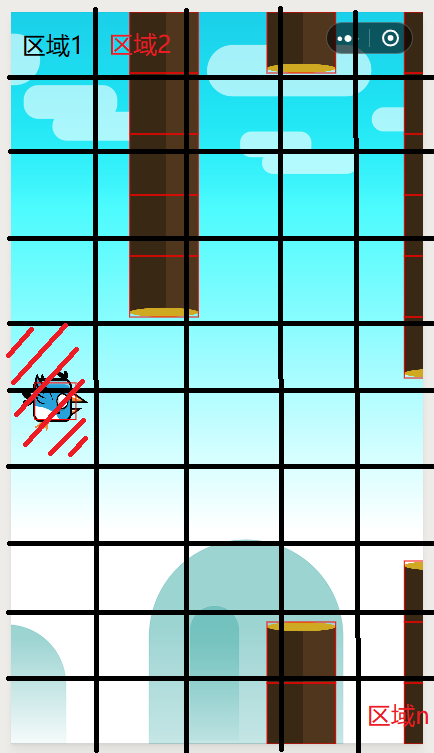updateFigureArea(figure);
}

function onFigureTransformChanged(figure){
updateFigureArea(figure);
}

function updateFigureArea(figure){
// 得到figure对应区域数组
let areas = findFigureAreas(figure);
// 清空figure维护的area数组
while(figure.areaArray.length !=0){
let areaId = figure.areaArray.pop();
let area = getArea(areaId);
area.remove(figure.id);
}
figure.areaArray.length = 0;// 清空figure所在区域id数组
// 重新设置一遍
while(areas.length !=0){
let area = areas.pop();
figure.areaArray.push(area.id);
}
}


let otherFigures = [];
for(let i = 0 ; i < bird.areaArray.length; i++){
let area = getArea(bird.areaArray[i]);
copyAll(otherFigures,area.figures);
}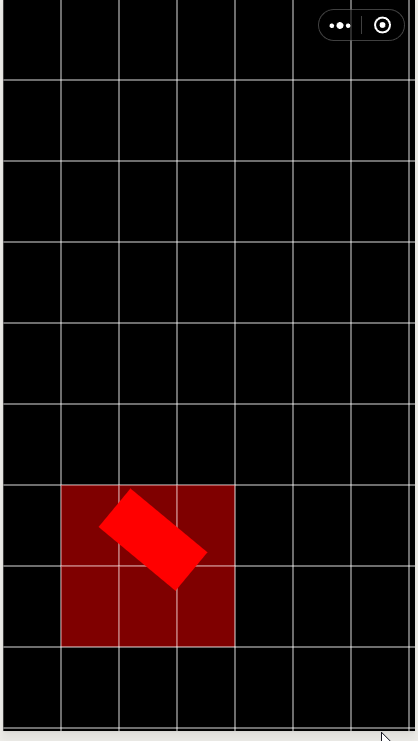#### devicePixelRatio

devicePixelRatio顾名思义，设备分辨比。

let systemInfo = wx.getSystemInfoSync();
let scale = systemInfo.pixelRatio;


let scale = windows.devicePixelRatio;
canvas.width *= scale;
cavans.height *= scale;


let scale = windows.devicePixelRatio;
canvas.style.width = canvas.width;// 微信小游戏没有style
cavnas.style.height = canvas.height;
canvas.width *= scale;
cavans.height *= scale;


// 之前这么写的
let myFigure.width = canvas.width;
myFigure.draw();
.....
// 后来才改的内存大小
let scale = windows.devicePixelRatio;
canvas.style.width = canvas.width; // 微信小游戏没有style
cavnas.style.height = canvas.height;
canvas.width *= scale;
cavans.height *= scale;
// 那就让ctx放大scale倍
let ctx = canvas.getContext('2d');
ctx.scale(scale,scale);


#### context 2d的clip方法少用为妙

https://developers.weixin.qq.com/s/AQNhhimW7A4s

#### 游戏不分贵贱